# Lecture 21 – Data 100, Fall 2020¶

Notebook by Fernando Pérez, Suraj Rampure, John DeNero, Sam Lau, Ani Adhikari.

In :
import numpy as np
import pandas as pd
import sklearn.linear_model as lm
from sklearn.datasets import load_boston

import matplotlib.pyplot as plt
import seaborn as sns
import plotly.express as px
import plotly.graph_objects as go
from tqdm.notebook import tnrange

plt.rcParams['figure.figsize'] = (4, 4)
plt.rcParams['figure.dpi'] = 150
plt.rcParams['lines.linewidth'] = 3
sns.set()


## Estimation and Bootstrapping¶

### Sample Mean Estimator¶

Let's say our population is finite and we know it: a uniform over the numbers 0 to 10,000 (inclusive). (Note: You would never need statistical inference if you knew the whole population; we're just creating a playground to try out techniques.)

In :
population = np.arange(10001)

In :
population

Out:
array([    0,     1,     2, ...,  9998,  9999, 10000])

We might want to know the population mean. In this case, we do!

In :
np.mean(population)

Out:
5000.0

But if we only had a sample, then we would perhaps estimate (guess) that the sample mean is a reasonable approximation for the true mean.

In :
sample_100 = np.random.choice(population, size=100, replace=False)
np.mean(sample_100)

Out:
4967.69

In this case, the estimator is the function np.mean and the population parameter is 5000. The estimate is close, but it's wrong.

### Sample variance estimator for the variance of the sample mean¶

Here's an impractical but effective method for estimating the variance of an estimator $f$. (Note that this process is not directly related to the true population parameter, we are instead trying to get a sense of how much our guesses vary from one another.)

In :
def var_estimate(f, pop, m=4000, n=100):
"""Estimate the variance of estimator f by the empirical variance.

f: A function of a sample
pop: An array representing the whole population
m, n: Use m samples of size n to estimate the variance
"""
estimates = []
for j in range(m):
sample = np.random.choice(pop, size=n, replace=False)
estimates.append(f(sample))
estimates = np.array(estimates)
plt.hist(estimates, bins=30)
plt.xlim(4000, 6000)
return np.var(estimates)

var_estimate(np.mean, population)

Out:
80223.9241133486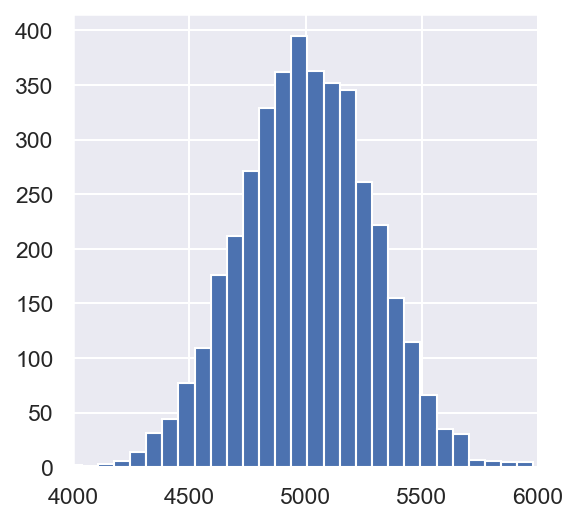In :
_**0.5

Out:
283.2382815110779
In :
var_estimate(np.mean, population, n=400)

Out:
19218.018342761905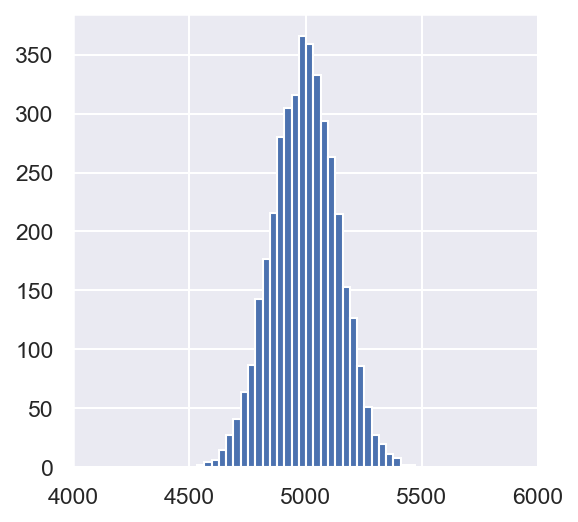In :
var_estimate(np.mean, population, n=1600)

Out:
4443.93657679553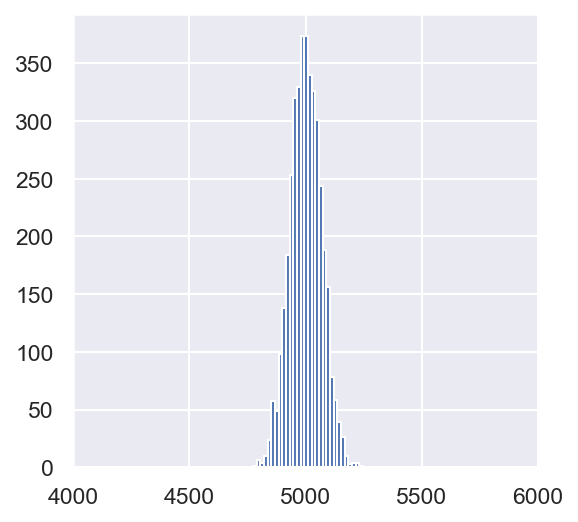This is not a new phenomenon. In Lecture 3, we saw that the variance of the sample mean decreases as our sample size increases.

If we know the variance of the sampling distribution and we know that the sampling distribution is approximately normal, then we know how far off a single estimate is likely to be. About 95% of estimates will be within 2 standard deviations of the mean, so for 95% of samples, the estimate will be off by the following (or less).

In :
2 * np.sqrt(var_estimate(np.mean, population))

Out:
573.5149473489605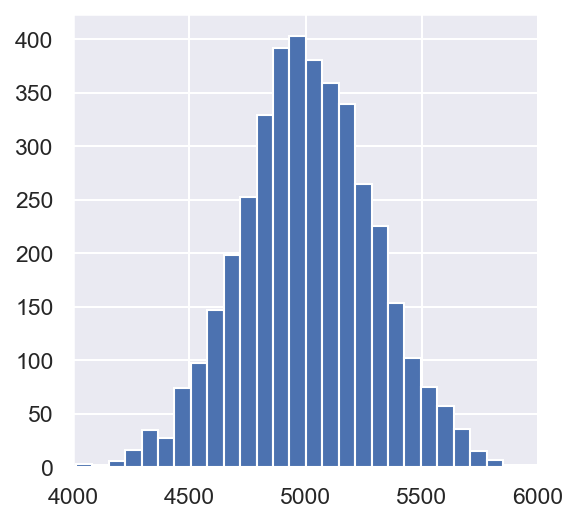Unfortunately, estimating the variance required repeated sampling from the population.

### Bootstrap estimator for the variance of the sample mean¶

Instead, we can estimate the variance using bootstrap resampling.

In :
def bootstrap_var_estimate(f, sample, m=4000):
"""Estimate the variance of estimator f by the empirical variance.

f: A function of a sample
sample: An array representing a sample of size n
m: Use m samples of size n to estimate the variance
"""
estimates = []
n = len(sample)
for j in range(m):
resample = np.random.choice(sample, size=n, replace=True)
estimates.append(f(resample))
estimates = np.array(estimates)
plt.hist(estimates, bins=30)
return np.mean((estimates - np.mean(estimates))**2) # same as np.var(estimates)

bootstrap_var_estimate(np.mean, sample_100)

Out:
89000.98314141778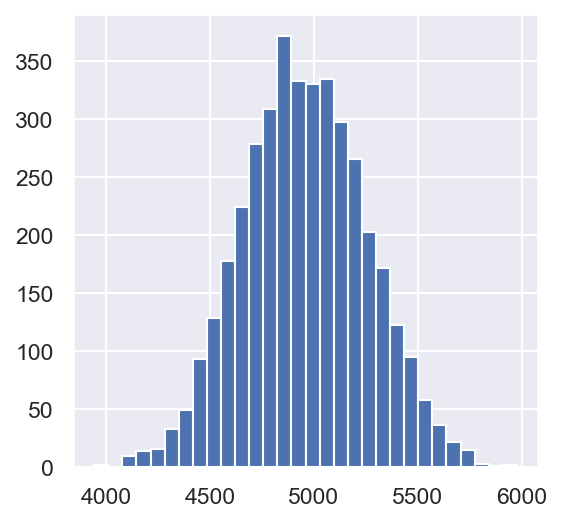In :
np.mean(sample_100)

Out:
4967.69
In :
sample_400 = np.random.choice(population, 400, replace=False)
bootstrap_var_estimate(np.mean, sample_400)

Out:
20391.037715224364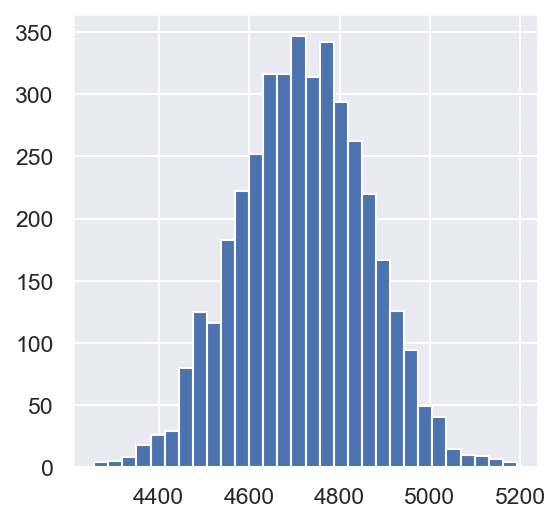In :
sample_1600 = np.random.choice(population, 1600, replace=False)
bootstrap_var_estimate(np.mean, sample_1600)

Out:
5256.434691224124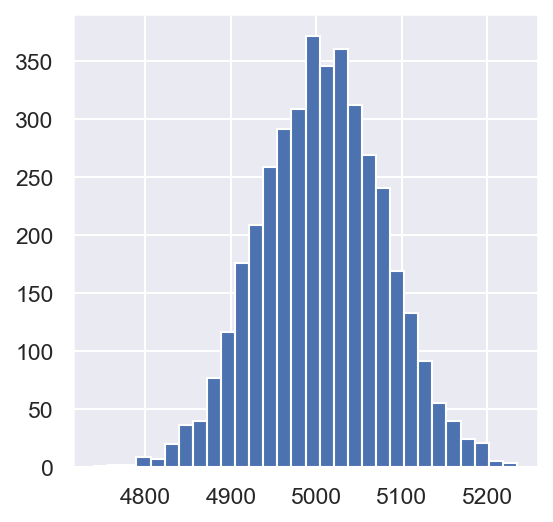We can see that the estimated variance when bootstrapping our sample is close to the variance computed by directly sampling from the population. But, it's a good amount wrong each time.

### Bootstrap confidence interval¶

In :
def ci(sample, estimator, confidence=95, m=1000):
"""Compute a confidence interval for an estimator.

sample: A DataFrame or Series
estimator: A function from a sample DataFrame to an estimate (number)
"""
if isinstance(sample, np.ndarray):
sample = pd.Series(sample)
estimates = []
n = sample.shape
for j in range(m):
resample = sample.sample(n, replace=True)
estimates.append(estimator(resample))
estimates = np.array(estimates)
slack = 100 - confidence
lower = np.percentile(estimates, slack/2)
upper = np.percentile(estimates, 100 - slack/2)
return (lower, upper)


Here's one bootstrapped confidence interval for the sample mean.

In :
np.mean(sample_100)

Out:
4967.69
In :
ci(sample_100, np.mean)

Out:
(4405.699, 5545.78625)
In :
def bootstrap_dist(sample, estimator, m=10000):
if isinstance(sample, np.ndarray):
sample = pd.Series(sample)
estimates = []
n = sample.shape
for j in range(m):
resample = sample.sample(n, replace=True)
estimates.append(estimator(resample))
plt.hist(estimates, bins=30)

bootstrap_dist(sample_100, np.mean)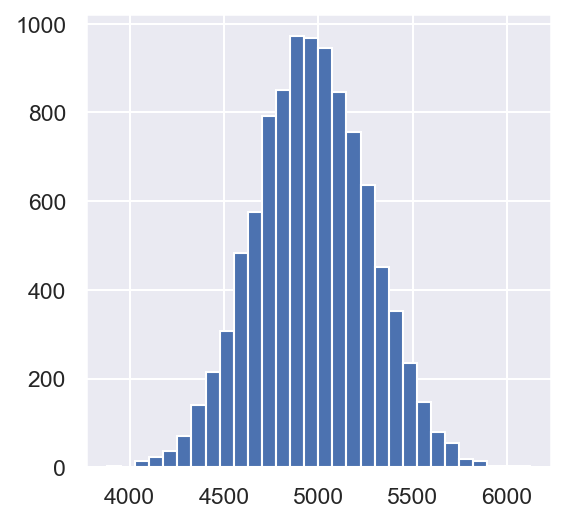To be crystal clear, the above histogram was computed by:

• resampling from our original sample sample_100, 10000 times
• each of those 10000 times, computing the sample mean
• calling plt.hist on the list of 10000 bootstrapped sample means

Let's create 100 95% confidence intervals for the sample mean. We'd expect roughly 95% of them to contain the true population parameter. In practice, we wouldn't be able to check (because if we knew the true population parameter, we wouldn't be doing any of this).

In :
mean_ints = [ci(np.random.choice(population, 100), np.mean)
for _ in tnrange(100)]


You will note, many of these intervals contain the true population parameter 5000, but some do not. Let's print the first few:

In :
mean_ints[:5]

Out:
[(4679.685750000001, 5836.7390000000005),
(4481.21225, 5608.8315),
(4372.71175, 5415.025000000001),
(4402.00475, 5441.0957499999995),
(4566.9172499999995, 5608.1542500000005)]

We can visualize this by plotting each confidence interval as a line and then overlaying the true mean, which we happen to know in this case is 5000. We can then count how many of our CIs include 5000. The code below does that, while also color-coding the CIs that fail to include the true value in red (the true mean is the thick vertical orange line).

We expect the fraction of "OK CIs" (that is, confidence intervals that include the true mean) to be close to 0.95, but the exact value you get will change each time you rerun the above code:

In :
true_mean = 5000
fig, ax = plt.subplots(figsize=(3,6))
nreps = len(mean_ints)
nok = 0
for i, bounds in enumerate(mean_ints):
if bounds <= true_mean <= bounds:
color = 'blue'
nok += 1
else:
color = 'red'
ax.plot(bounds, [i, i], '-o', color=color, lw=1, markersize=2)
ax.axvline(true_mean, color='orange', lw=3);
ax.set_title(f"Fraction of OK CIs: {nok/nreps}");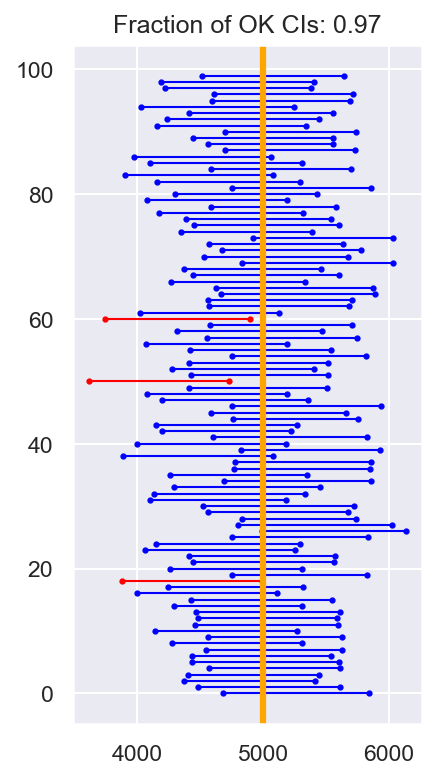### Bootstrap confidence intervals for other population parameters¶

#### Median¶

In :
bootstrap_dist(sample_100, np.median)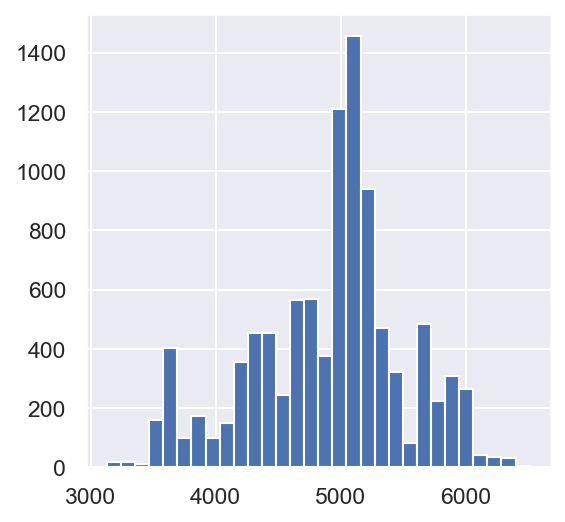In :
ci(sample_100, np.median)

Out:
(3629.5, 5996.0)
In :
# True population median
np.median(population)

Out:
5000.0

#### Standard Deviation¶

In :
bootstrap_dist(sample_100, np.std)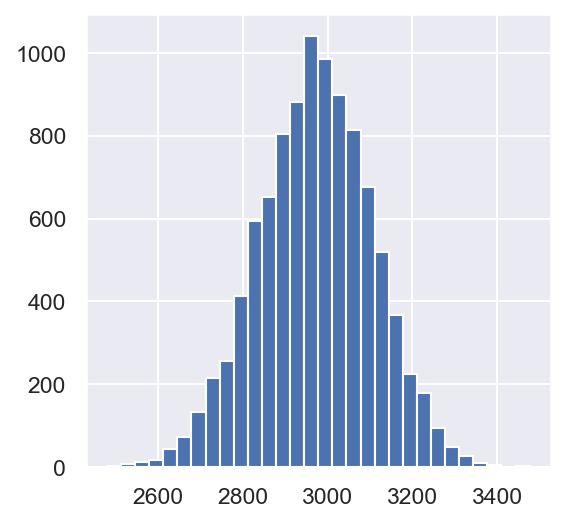In :
ci(sample_100, np.std)

Out:
(2716.0975023629376, 3230.2375200109923)
In :
# True population standard deviation
np.std(population)

Out:
2887.0400066504103

#### 99th Percentile¶

In :
p99 = lambda a: np.percentile(a, 99)
p99(population)

Out:
9900.0
In :
bootstrap_dist(sample_100, p99)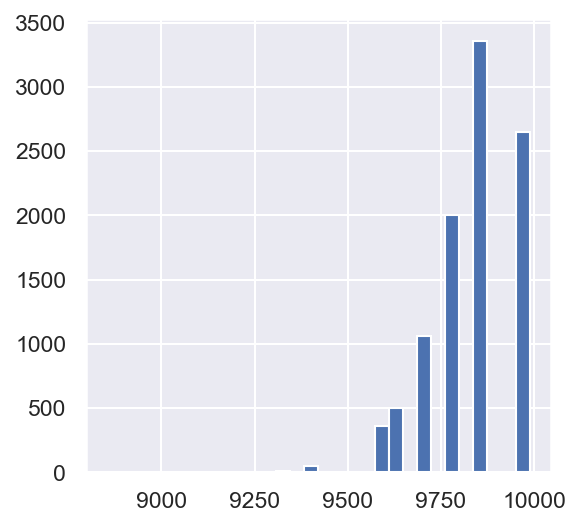In :
ci(sample_100, p99)

Out:
(9587.16, 9988.0)
In :
p99_ints = [ci(np.random.choice(population, 100), p99)
for _ in tnrange(100)]


In :
sum([v <= p99(population) <= v for v in p99_ints])

Out:
68

Extreme percentiles aren't estimated well with the bootstrap. Only 60-70 / 100 of our 95% confidence intervals contained the true population parameter.

## Estimating Parameters in Linear Regression¶

Let's revisit an old friend.

In :
nba = pd.read_csv('nba18-19.csv')

In :
nba

Out:
Rk Player Pos Age Tm G GS MP FG FGA ... FT% ORB DRB TRB AST STL BLK TOV PF PTS
0 1 Álex Abrines\abrinal01 SG 25 OKC 31 2 19.0 1.8 5.1 ... 0.923 0.2 1.4 1.5 0.6 0.5 0.2 0.5 1.7 5.3
1 2 Quincy Acy\acyqu01 PF 28 PHO 10 0 12.3 0.4 1.8 ... 0.700 0.3 2.2 2.5 0.8 0.1 0.4 0.4 2.4 1.7
2 3 Jaylen Adams\adamsja01 PG 22 ATL 34 1 12.6 1.1 3.2 ... 0.778 0.3 1.4 1.8 1.9 0.4 0.1 0.8 1.3 3.2
3 4 Steven Adams\adamsst01 C 25 OKC 80 80 33.4 6.0 10.1 ... 0.500 4.9 4.6 9.5 1.6 1.5 1.0 1.7 2.6 13.9
4 5 Bam Adebayo\adebaba01 C 21 MIA 82 28 23.3 3.4 5.9 ... 0.735 2.0 5.3 7.3 2.2 0.9 0.8 1.5 2.5 8.9
... ... ... ... ... ... ... ... ... ... ... ... ... ... ... ... ... ... ... ... ... ...
703 528 Tyler Zeller\zellety01 C 29 MEM 4 1 20.5 4.0 7.0 ... 0.778 2.3 2.3 4.5 0.8 0.3 0.8 1.0 4.0 11.5
704 529 Ante Žižić\zizican01 C 22 CLE 59 25 18.3 3.1 5.6 ... 0.705 1.8 3.6 5.4 0.9 0.2 0.4 1.0 1.9 7.8
705 530 Ivica Zubac\zubaciv01 C 21 TOT 59 37 17.6 3.6 6.4 ... 0.802 1.9 4.2 6.1 1.1 0.2 0.9 1.2 2.3 8.9
706 530 Ivica Zubac\zubaciv01 C 21 LAL 33 12 15.6 3.4 5.8 ... 0.864 1.6 3.3 4.9 0.8 0.1 0.8 1.0 2.2 8.5
707 530 Ivica Zubac\zubaciv01 C 21 LAC 26 25 20.2 3.8 7.2 ... 0.733 2.3 5.3 7.7 1.5 0.4 0.9 1.4 2.5 9.4

708 rows × 30 columns

This table provides aggregate statistics for each player throughout the 2018-19 NBA season.

Let's use FG, FGA, FT%, 3PA, and AST to predict PTS. For reference:

• FG is the number of shots a player made.
• FGA is the number of shots a player took.
• FT% is the proportion of free throw shots a player took that they made.
• 3PA is the number of three-point shots a player took.
• AST is the number of assists the player had.
In :
nba_small = nba[['FG', 'FGA', 'FT%', '3PA', 'AST', 'PTS']].fillna(0)
nba_small

Out:
FG FGA FT% 3PA AST PTS
0 1.8 5.1 0.923 4.1 0.6 5.3
1 0.4 1.8 0.700 1.5 0.8 1.7
2 1.1 3.2 0.778 2.2 1.9 3.2
3 6.0 10.1 0.500 0.0 1.6 13.9
4 3.4 5.9 0.735 0.2 2.2 8.9
... ... ... ... ... ... ...
703 4.0 7.0 0.778 0.0 0.8 11.5
704 3.1 5.6 0.705 0.0 0.9 7.8
705 3.6 6.4 0.802 0.0 1.1 8.9
706 3.4 5.8 0.864 0.0 0.8 8.5
707 3.8 7.2 0.733 0.0 1.5 9.4

708 rows × 6 columns

Note that this is really just for the sake of example; the correlation between FG and PTS is so high that we in practice wouldn't need all of these other features.

In :
reg = lm.LinearRegression()
reg.fit(nba_small.iloc[:,:-1], nba_small.iloc[:,-1])

Out:
LinearRegression()

The Multiple $R^2$ value is quite high:

In :
reg.score(nba_small.iloc[:,:-1], nba_small.iloc[:,-1])

Out:
0.9886417979129902

Let's look at the coefficients, though:

In :
nba_small.columns

Out:
Index(['FG', 'FGA', 'FT%', '3PA', 'AST', 'PTS'], dtype='object')
In :
reg.coef_.astype(float)

Out:
array([2.44566707, 0.03633589, 0.50454007, 0.28367305, 0.04144433])

The coefficient on FGA, the number of shots a player takes, is very low. This means that FGA is not very useful in predicting PTS. That's strange – because we'd think that the number of shots a player takes would be very useful in such a case.

Let's look at a 95% confidence interval (created using the bootstrap percentile technique from above) for the coefficient on FGA.

In :
def FGA_slope(t):
reg = lm.LinearRegression().fit(t.iloc[:,:-1], t.iloc[:,-1])
return reg.coef_

ci(nba_small, FGA_slope)

Out:
(-0.06721086163736958, 0.13469964057818726)

We see 0 is in this interval. Hmmmm....

## Multicollinearity¶

The issue is that FGA is highly correlated with one of the other features in our design matrix.

In :
nba_small.corr()

Out:
FG FGA FT% 3PA AST PTS
FG 1.000000 0.973355 0.371598 0.600830 0.665761 0.990014
FGA 0.973355 1.000000 0.395902 0.725114 0.703093 0.980447
FT% 0.371598 0.395902 1.000000 0.377633 0.288057 0.401555
3PA 0.600830 0.725114 0.377633 1.000000 0.480880 0.666673
AST 0.665761 0.703093 0.288057 0.480880 1.000000 0.676022
PTS 0.990014 0.980447 0.401555 0.666673 0.676022 1.000000

The correlation between FGA and FG is very close to 1. This is a sign of multicollinearity, meaning that the individual coefficients have little meaning.

Let's look at the resulting model that comes from only using FGA as a feature.

In :
simple_model = lm.LinearRegression()
simple_model.fit(nba_small[['FGA']].values.reshape(-1, 1), nba_small['PTS'])

Out:
LinearRegression()

We dropped all of those features, but the Multiple $R^2$ value is almost the same. The simpler, the better.

In :
simple_model.score(nba_small[['FGA']].values.reshape(-1, 1), nba_small['PTS'])

Out:
0.9612756271235542

The coefficient on FGA in this model, of course, is positive.

In :
simple_model.coef_

Out:
array([1.29982787])
In :
def FGA_slope_simple_model(t):
reg = lm.LinearRegression().fit(t[['FGA']].values.reshape(-1, 1), t['PTS'])
return reg.coef_

ci(nba_small, FGA_slope_simple_model)

Out:
(1.2738104997633222, 1.3284630654931016)

0 is not in this interval, so we know that the slope for FGA in the linear model with PTS is significantly different than 0.